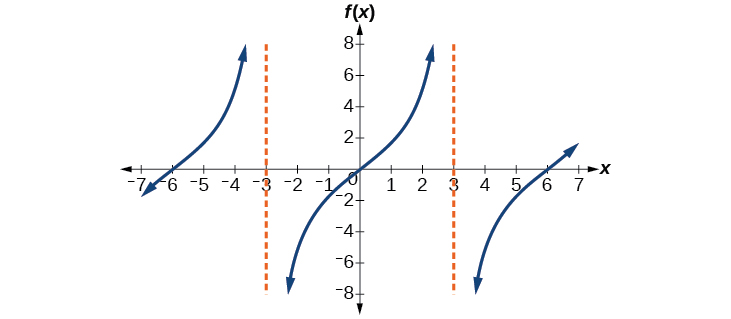# 8.2 Graphs of the other trigonometric functions  (Page 2/9)

 Page 2 / 9

## Graphing variations of y = tan x

As with the sine and cosine functions, the tangent    function can be described by a general equation.

$y=A\mathrm{tan}\left(Bx\right)$

We can identify horizontal and vertical stretches and compressions using values of $\text{\hspace{0.17em}}A\text{\hspace{0.17em}}$ and $\text{\hspace{0.17em}}B.\text{\hspace{0.17em}}$ The horizontal stretch can typically be determined from the period of the graph. With tangent graphs, it is often necessary to determine a vertical stretch using a point on the graph.

Because there are no maximum or minimum values of a tangent function, the term amplitude cannot be interpreted as it is for the sine and cosine functions. Instead, we will use the phrase stretching/compressing factor when referring to the constant $\text{\hspace{0.17em}}A.$

## Features of the graph of y = A Tan( Bx )

• The stretching factor is $\text{\hspace{0.17em}}|A|.$
• The period is $\text{\hspace{0.17em}}P=\frac{\pi }{|B|}.$
• The domain is all real numbers $\text{\hspace{0.17em}}x,$ where $\text{\hspace{0.17em}}x\ne \frac{\pi }{2|B|}+\frac{\pi }{|B|}k\text{\hspace{0.17em}}$ such that $\text{\hspace{0.17em}}k\text{\hspace{0.17em}}$ is an integer.
• The range is $\text{\hspace{0.17em}}\left(\mathrm{-\infty },\infty \right).$
• The asymptotes occur at $\text{\hspace{0.17em}}x=\frac{\pi }{2|B|}+\frac{\pi }{|B|}k,\text{\hspace{0.17em}}$ where $\text{\hspace{0.17em}}k\text{\hspace{0.17em}}$ is an integer.
• $y=A\mathrm{tan}\left(Bx\right)\text{\hspace{0.17em}}$ is an odd function.

## Graphing one period of a stretched or compressed tangent function

We can use what we know about the properties of the tangent function to quickly sketch a graph of any stretched and/or compressed tangent function of the form $\text{\hspace{0.17em}}f\left(x\right)=A\mathrm{tan}\left(Bx\right).\text{\hspace{0.17em}}$ We focus on a single period    of the function including the origin, because the periodic property enables us to extend the graph to the rest of the function’s domain if we wish. Our limited domain is then the interval $\text{\hspace{0.17em}}\left(-\frac{P}{2},\frac{P}{2}\right)\text{\hspace{0.17em}}$ and the graph has vertical asymptotes at $\text{\hspace{0.17em}}±\frac{P}{2}\text{\hspace{0.17em}}$ where $\text{\hspace{0.17em}}P=\frac{\pi }{B}.\text{\hspace{0.17em}}$ On $\text{\hspace{0.17em}}\left(-\frac{\pi }{2},\frac{\pi }{2}\right),\text{\hspace{0.17em}}$ the graph will come up from the left asymptote at $\text{\hspace{0.17em}}x=-\frac{\pi }{2},\text{\hspace{0.17em}}$ cross through the origin, and continue to increase as it approaches the right asymptote at $\text{\hspace{0.17em}}x=\frac{\pi }{2}.\text{\hspace{0.17em}}$ To make the function approach the asymptotes at the correct rate, we also need to set the vertical scale by actually evaluating the function for at least one point that the graph will pass through. For example, we can use

$f\left(\frac{P}{4}\right)=A\mathrm{tan}\left(B\frac{P}{4}\right)=A\mathrm{tan}\left(B\frac{\pi }{4B}\right)=A$

because $\text{\hspace{0.17em}}\mathrm{tan}\left(\frac{\pi }{4}\right)=1.$

Given the function $\text{\hspace{0.17em}}f\left(x\right)=A\mathrm{tan}\left(Bx\right),\text{\hspace{0.17em}}$ graph one period.

1. Identify the stretching factor, $\text{\hspace{0.17em}}|A|.$
2. Identify $\text{\hspace{0.17em}}B\text{\hspace{0.17em}}$ and determine the period, $\text{\hspace{0.17em}}P=\frac{\pi }{|B|}.$
3. Draw vertical asymptotes at $\text{\hspace{0.17em}}x=-\frac{P}{2}\text{\hspace{0.17em}}$ and $\text{\hspace{0.17em}}x=\frac{P}{2}.$
4. For $\text{\hspace{0.17em}}A>0,\text{\hspace{0.17em}}$ the graph approaches the left asymptote at negative output values and the right asymptote at positive output values (reverse for $\text{\hspace{0.17em}}A<0$ ).
5. Plot reference points at $\text{\hspace{0.17em}}\left(\frac{P}{4},A\right),\text{\hspace{0.17em}}$ $\left(0,0\right),\text{\hspace{0.17em}}$ and $\text{\hspace{0.17em}}\left(-\frac{P}{4},-A\right),\text{\hspace{0.17em}}$ and draw the graph through these points.

## Sketching a compressed tangent

Sketch a graph of one period of the function $\text{\hspace{0.17em}}y=0.5\mathrm{tan}\left(\frac{\pi }{2}x\right).$

First, we identify $\text{\hspace{0.17em}}A\text{\hspace{0.17em}}$ and $\text{\hspace{0.17em}}B.$Because $\text{\hspace{0.17em}}A=0.5\text{\hspace{0.17em}}$ and $\text{\hspace{0.17em}}B=\frac{\pi }{2},\text{\hspace{0.17em}}$ we can find the stretching/compressing factor and period. The period is $\text{\hspace{0.17em}}\frac{\pi }{\frac{\pi }{2}}=2,\text{\hspace{0.17em}}$ so the asymptotes are at $\text{\hspace{0.17em}}x=±1.\text{\hspace{0.17em}}$ At a quarter period from the origin, we have

$\begin{array}{l}f\left(0.5\right)=0.5\mathrm{tan}\left(\frac{0.5\pi }{2}\right)\hfill \\ \text{\hspace{0.17em}}\text{\hspace{0.17em}}\text{\hspace{0.17em}}\text{\hspace{0.17em}}\text{\hspace{0.17em}}\text{\hspace{0.17em}}\text{\hspace{0.17em}}\text{\hspace{0.17em}}\text{\hspace{0.17em}}\text{\hspace{0.17em}}\text{\hspace{0.17em}}\text{\hspace{0.17em}}\text{\hspace{0.17em}}\text{\hspace{0.17em}}\text{\hspace{0.17em}}=0.5\mathrm{tan}\left(\frac{\pi }{4}\right)\hfill \\ \text{\hspace{0.17em}}\text{\hspace{0.17em}}\text{\hspace{0.17em}}\text{\hspace{0.17em}}\text{\hspace{0.17em}}\text{\hspace{0.17em}}\text{\hspace{0.17em}}\text{\hspace{0.17em}}\text{\hspace{0.17em}}\text{\hspace{0.17em}}\text{\hspace{0.17em}}\text{\hspace{0.17em}}\text{\hspace{0.17em}}\text{\hspace{0.17em}}\text{\hspace{0.17em}}=0.5\hfill \end{array}$

This means the curve must pass through the points $\text{\hspace{0.17em}}\left(0.5,0.5\right),$ $\left(0,0\right),$ and $\text{\hspace{0.17em}}\left(-0.5,-0.5\right).\text{\hspace{0.17em}}$ The only inflection point is at the origin. [link] shows the graph of one period of the function.

Sketch a graph of $\text{\hspace{0.17em}}f\left(x\right)=3\mathrm{tan}\left(\frac{\pi }{6}x\right).$## Graphing one period of a shifted tangent function

Now that we can graph a tangent function that is stretched or compressed, we will add a vertical and/or horizontal (or phase) shift. In this case, we add $\text{\hspace{0.17em}}C\text{\hspace{0.17em}}$ and $\text{\hspace{0.17em}}D\text{\hspace{0.17em}}$ to the general form of the tangent function.

#### Questions & Answers

find to nearest one decimal place of centimeter the length of an arc of circle of radius length 12.5cm and subtending of centeral angle 1.6rad
factoring polynomial
find general solution of the Tanx=-1/root3,secx=2/root3
find general solution of the following equation
Nani
the value of 2 sin square 60 Cos 60
0.75
Lynne
0.75
Inkoom
when can I use sin, cos tan in a giving question
depending on the question
Nicholas
I am a carpenter and I have to cut and assemble a conventional roof line for a new home. The dimensions are: width 30'6" length 40'6". I want a 6 and 12 pitch. The roof is a full hip construction. Give me the L,W and height of rafters for the hip, hip jacks also the length of common jacks.
John
I want to learn the calculations
where can I get indices
I need matrices
Nasasira
hi
Raihany
Hi
Solomon
need help
Raihany
maybe provide us videos
Nasasira
Raihany
Hello
Cromwell
a
Amie
What do you mean by a
Cromwell
nothing. I accidentally press it
Amie
you guys know any app with matrices?
Khay
Ok
Cromwell
Solve the x? x=18+(24-3)=72
x-39=72 x=111
Suraj
Solve the formula for the indicated variable P=b+4a+2c, for b
Need help with this question please
b=-4ac-2c+P
Denisse
b=p-4a-2c
Suddhen
b= p - 4a - 2c
Snr
p=2(2a+C)+b
Suraj
b=p-2(2a+c)
Tapiwa
P=4a+b+2C
COLEMAN
b=P-4a-2c
COLEMAN
like Deadra, show me the step by step order of operation to alive for b
John
A laser rangefinder is locked on a comet approaching Earth. The distance g(x), in kilometers, of the comet after x days, for x in the interval 0 to 30 days, is given by g(x)=250,000csc(π30x). Graph g(x) on the interval [0, 35]. Evaluate g(5)  and interpret the information. What is the minimum distance between the comet and Earth? When does this occur? To which constant in the equation does this correspond? Find and discuss the meaning of any vertical asymptotes.
The sequence is {1,-1,1-1.....} has
circular region of radious
how can we solve this problem
Sin(A+B) = sinBcosA+cosBsinA
Prove it
Eseka
Eseka
hi
Joel
yah
immyByByByByBy Nick Swain